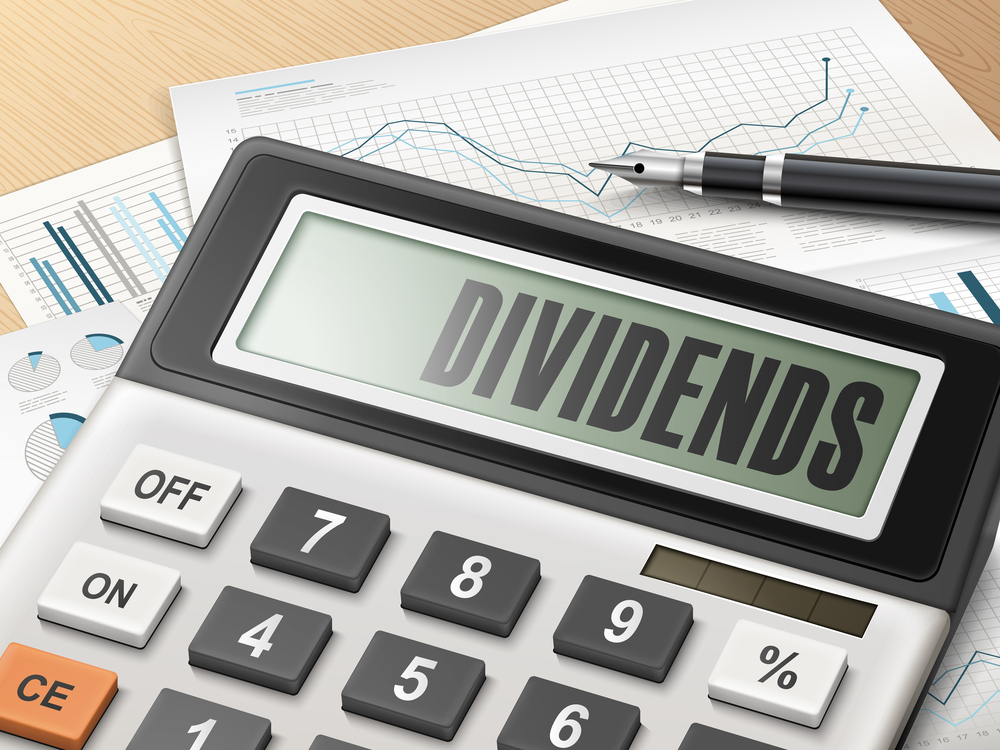Continue to site >
Trending ETFsDividend University

# Valuing a Non-dividend Paying Company with the Dividend Discount Model

We saw how we can find out the present value of a dividend paying company here. We also saw how dividend paying companies are valued using multiple growth stage models with the H-Model, the Two-Stage Dividend Model, and the Three-Stage Dividend Model.

The basic formula that we use is:
Stock Value = D1/r-G

In this equation, D1 is the expected dividend payment one year from the current date, r is the required rate of return, and G is the dividend growth rate expected to continue in perpetuity.

However, this equation can also be applied to stocks that don’t pay a dividend. The same principles of discounting the dividend back can be applied by assuming that the company may not pay a dividend right now, but may start paying a dividend a few years down the line. Generally when we value non-dividend paying stocks using the DDM model, we use a multistage model where we assume that in the first stage, the dividend paid out would be zero.

## Example

Assume that ABC Inc hasn’t paid a dividend and is not expected to pay one in the near future. If the company starts paying a dividend of \$1 five years from now and is expected to grow at 5% from then, this future dividend stream can be discounted back using the dividend discount model. Assume that the discount rate of the company is 11%.

Value of the stock four years from now will be V4 = D5 / r-g
I.e. 1/(0.11-0.05) = \$16.67

Now when you find the value of the stock today, we discount the value we found four years from now.
V0 = V4/(1+r)^4 = \$10.98

The fair value of the stock is \$10.98. If the current market price of the stock is greater than \$10.98 then the stock is a “sell,” and if the current market price of the stock is less than \$10.98 then it’s a “buy.”

## The Bottom Line

The method mentioned above is not the best method to value non-dividend paying stocks. It’s extremely difficult for an investor to guess how much the dividend initiation could be and after how many years it will occur. It’s difficult to measure the growth rate after the dividend initiation, which increases the modelling risk while inputting numbers in the formula.

The best method to analyze such stocks would be the free cash flow based model or the residual income based model.